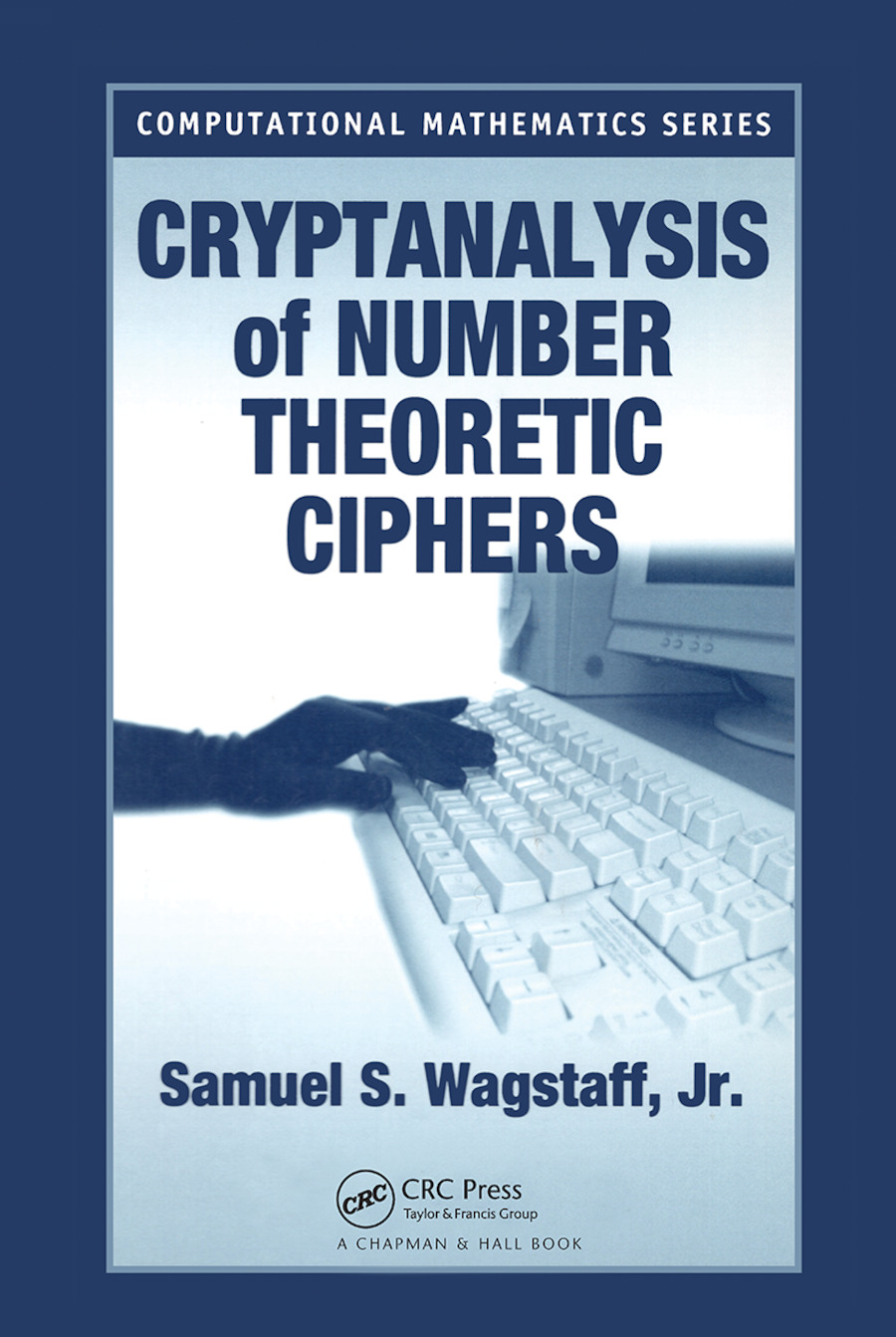Cryptanalysis of Number Theoretic Ciphers

1st Edition

Chapman and Hall/CRC

336 pages | 7 B/W Illus.

Hardback: 9781584881537
pub: 2002-12-10
Currently out of stock
\$135.00
x

FREE Standard Shipping!

Description

At the heart of modern cryptographic algorithms lies computational number theory. Whether you're encrypting or decrypting ciphers, a solid background in number theory is essential for success. Written by a number theorist and practicing cryptographer, Cryptanalysis of Number Theoretic Ciphers takes you from basic number theory to the inner workings of ciphers and protocols.

First, the book provides the mathematical background needed in cryptography as well as definitions and simple examples from cryptography. It includes summaries of elementary number theory and group theory, as well as common methods of finding or constructing large random primes, factoring large integers, and computing discrete logarithms. Next, it describes a selection of cryptographic algorithms, most of which use number theory. Finally, the book presents methods of attack on the cryptographic algorithms and assesses their effectiveness. For each attack method the author lists the systems it applies to and tells how they may be broken with it.

Computational number theorists are some of the most successful cryptanalysts against public key systems. Cryptanalysis of Number Theoretic Ciphers builds a solid foundation in number theory and shows you how to apply it not only when breaking ciphers, but also when designing ones that are difficult to break.

Reviews

"This book contains a nice introduction to modern cryptography, with emphasis laid on the ciphers based on computational number theory. … The reader learns to deal with large numbers in a computer and to analyze the complexity of the most basic algorithms. … [T]he reader is not just confronted with a list of algorithms, but he/she is given the clues to assimilate the ideas behind the results … . The book contains more than 200 interesting exercises that test the reader's understanding of the text."

- Zentralblatt MATH, 1045

"The book provides an excellent text concerning cryptanalysis. … masterly and carefully written. As the proofs are given with full details … I can recommend it also to students."

- Monatshefte fur Mathematik

MATHEMATICAL FOUNDATIONS OF CRYPTANALYSIS

Terminology of Cryptography

Probability Theory

Divisibility and Arithmetic

Primes

Congruences

Euler's Theorem and Its Consequences

Second Degree Congruences

Information Theory

Groups, Rings and Fields

Finding Large Primes

Exponential Methods of Factoring Integers

Elliptic Curves

Subexponential Factoring Algorithms

Computing Discrete Logarithms

Random Number Generation

THE CRYPTOGRAPHIC ALGORITHMS

Private Key Algorithms

Public Key Algorithms

Signature Algorithms

Key Exchange Algorithms

Simple Protocols

Complicated Protocols

Complete Systems

METHODS OF ATTACK

Direct Attacks

Exploiting a User Error

Active Attacks

References